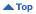Educational
HomeEducationalThe Sun and Solar ActivityGeneral InfoThe Source of Solar Energy Sunday, May 31 2020 07:01 UT

# Section Information

The Sun and Solar Activity

# The Source of Solar Energy

The source of all energy radiated by the Sun lies in its core, a central region comprising only 1.5% of the total solar volume. This is a very large thermonuclear reactor where Hydrogen (H) atoms are fused together to form Helium (He), releasing energy at the rate of 3.86 x 1026 joules per second. The fusion reactions in the solar core take place because of the very high temperatures and very large pressures present in this region of the Sun. Although there is no way of measuring these quantities directly, physical models of the Sun suggest that the core temperature is around 15 million degrees and the central pressure is about 250 billion atmospheres (250 billion times the pressure on the Earth at sea level).

It is the massive gravity of the Sun that compresses the core to such a high pressure and resultant high temperature, which then is sufficient to ignite the fusion reactions which take place. The overall result is to convert four hydrogen atoms into one helium atom (see below). For every one kilogram of hydrogen that is consumed, most is turned into helium but a small portion, 0.007 kg, is turned into pure energy. Using the famous energy-mass equivalence formula (E = mc2) developed by Einstein, we can calculate that this mass amounts to a little over 600 trillion joules (6 x 1014 J). When related to the total energy output of the Sun, this means that the solar fusion reactions are consuming mass at almost 5 million tonnes per second!

There are two distinct reactions in which four hydrogen atoms may eventually result in one helium atom. The first of these is:

(1) 1H + 1H → 2D + e+ + ν
then 2D + 1H → 3He + ɣ
then 3He + 3He → 4He + 1H + 1H

This reaction sequence is believed to be the most important one in the solar core. The total energy released by these reactions in turning four hydrogen atoms into one helium atom is 26.7 MeV.

The second reaction generates less than 10% of the total solar energy. This involves Carbon atoms (C) which are not consumed in the overall process. The details of this "carbon cycle" are as follows:

(2) 12C + 1H → 13N + ɣ
then 13N → 13C + e+ + ν
then 13C + 1H → 14N + ɣ
then 14N + 1H → 15O + ɣ
then 15O → 15N + e+ + ν
then 15N + 1H → 12C + 4He + ɣ

All the energy that is generated in the solar core escapes mostly in the form of very high energy gamma rays. This energy is absorbed and re-emitted many many times by the layers overlying the core, as the photons (bits of electromagnetic energy) diffuse out toward the surface. In doing so, the energy is degraded; gamma photons are turned into X-ray photons, and then into UV photons, and finally into visible light and infrared photons. And so it is light and heat that is finally radiated from the surface of the Sun into interplanetary space. This same heat and light has a flux density of 1370 watts per square metre by the time it arrives at the upper atmosphere of the Earth. It is this energy that makes life possible on the surface of the Earth, that produces our terrestrial weather, and that photovoltaic cells can convert into electrical energy. Solar energy is, in actuality, nuclear energy!

Material prepared by John Kennewell and Andrew McDonald